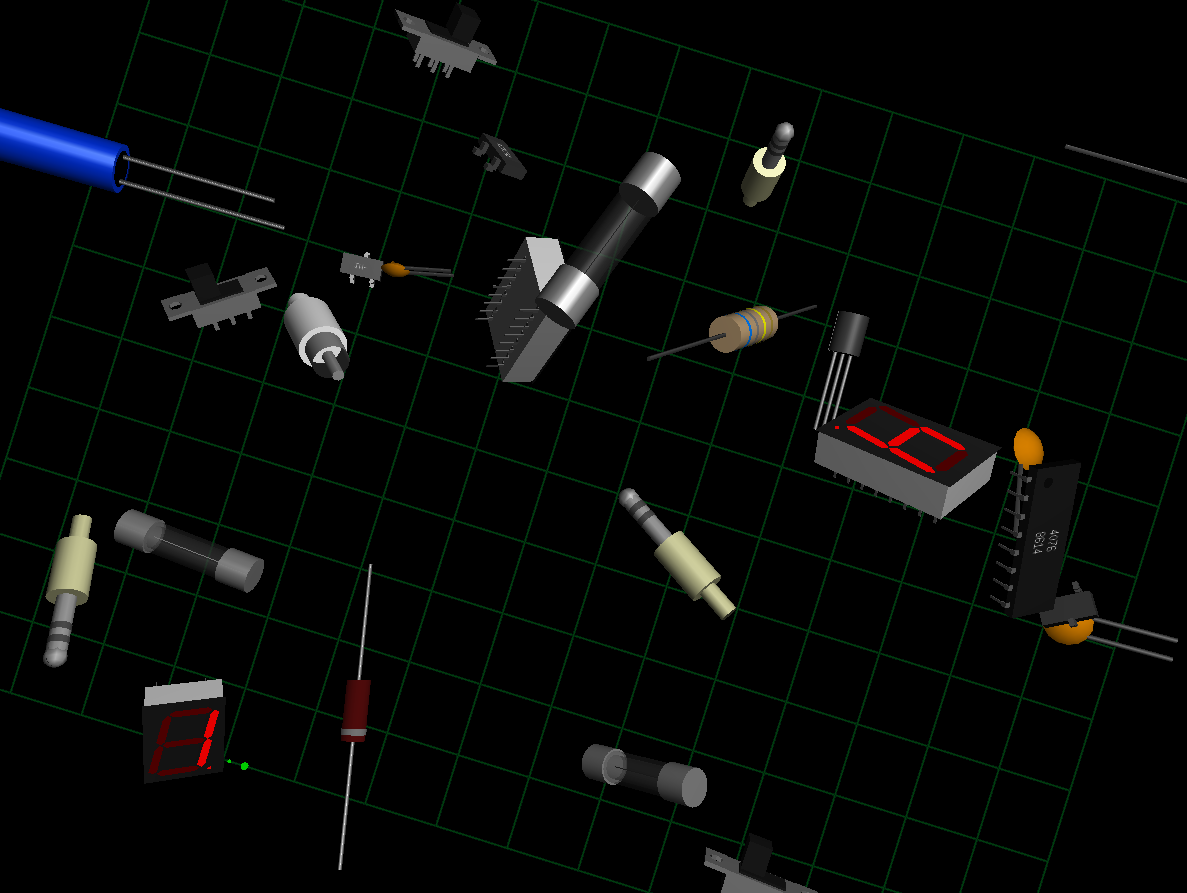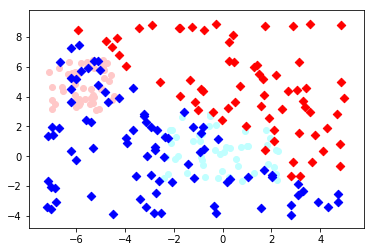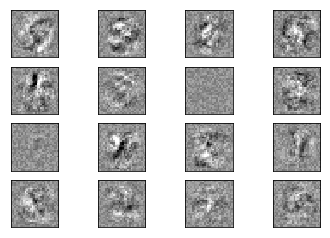## Neural Networks with scikit

### Perceptron ClassWe will start with the Perceptron class contained in Scikit-Learn. We will use it on the iris dataset, which we had already used in our chapter on k-nearest neighbor

import numpy as np
from sklearn.datasets import load_iris
from sklearn.linear_model import Perceptron
iris = load_iris()
print(iris.data[:3])
print(iris.data[15:18])
print(iris.data[37:40])
# we extract only the lengths and widthes of the petals:
X = iris.data[:, (2, 3)]

[[5.1 3.5 1.4 0.2]
[4.9 3.  1.4 0.2]
[4.7 3.2 1.3 0.2]]
[[5.7 4.4 1.5 0.4]
[5.4 3.9 1.3 0.4]
[5.1 3.5 1.4 0.3]]
[[4.9 3.6 1.4 0.1]
[4.4 3.  1.3 0.2]
[5.1 3.4 1.5 0.2]]


iris.label contains the labels 0, 1 and 2 corresponding three species of Iris flower:

• Iris setosa,
• Iris virginica and
• Iris versicolor.
print(iris.target)

[0 0 0 0 0 0 0 0 0 0 0 0 0 0 0 0 0 0 0 0 0 0 0 0 0 0 0 0 0 0 0 0 0 0 0 0 0
0 0 0 0 0 0 0 0 0 0 0 0 0 1 1 1 1 1 1 1 1 1 1 1 1 1 1 1 1 1 1 1 1 1 1 1 1
1 1 1 1 1 1 1 1 1 1 1 1 1 1 1 1 1 1 1 1 1 1 1 1 1 1 2 2 2 2 2 2 2 2 2 2 2
2 2 2 2 2 2 2 2 2 2 2 2 2 2 2 2 2 2 2 2 2 2 2 2 2 2 2 2 2 2 2 2 2 2 2 2 2
2 2]


We turn the three classes into two classes, i.e.

• Iris setosa
• not Iris setosa (this means Iris virginica or Iris versicolor)
y = (iris.target==0).astype(np.int8)
print(y)

[1 1 1 1 1 1 1 1 1 1 1 1 1 1 1 1 1 1 1 1 1 1 1 1 1 1 1 1 1 1 1 1 1 1 1 1 1
1 1 1 1 1 1 1 1 1 1 1 1 1 0 0 0 0 0 0 0 0 0 0 0 0 0 0 0 0 0 0 0 0 0 0 0 0
0 0 0 0 0 0 0 0 0 0 0 0 0 0 0 0 0 0 0 0 0 0 0 0 0 0 0 0 0 0 0 0 0 0 0 0 0
0 0 0 0 0 0 0 0 0 0 0 0 0 0 0 0 0 0 0 0 0 0 0 0 0 0 0 0 0 0 0 0 0 0 0 0 0
0 0]


We create now a Perceptron and fit the data X and y:

p = Perceptron(random_state=42,
max_iter=10,
tol=0.001)
p.fit(X, y)

The Python code above returned the following:
Perceptron(alpha=0.0001, class_weight=None, early_stopping=False, eta0=1.0,
fit_intercept=True, max_iter=10, n_iter=None, n_iter_no_change=5,
n_jobs=None, penalty=None, random_state=42, shuffle=True, tol=0.001,
validation_fraction=0.1, verbose=0, warm_start=False)

Now, we are ready for predictions:

values = [[1.5, 0.1], [1.8, 0.4], [1.3,0.2]]
for value in X:
pred = p.predict([value])
print([pred])

[array(, dtype=int8)]
[array(, dtype=int8)]
[array(, dtype=int8)]
[array(, dtype=int8)]
[array(, dtype=int8)]
[array(, dtype=int8)]
[array(, dtype=int8)]
[array(, dtype=int8)]
[array(, dtype=int8)]
[array(, dtype=int8)]
[array(, dtype=int8)]
[array(, dtype=int8)]
[array(, dtype=int8)]
[array(, dtype=int8)]
[array(, dtype=int8)]
[array(, dtype=int8)]
[array(, dtype=int8)]
[array(, dtype=int8)]
[array(, dtype=int8)]
[array(, dtype=int8)]
[array(, dtype=int8)]
[array(, dtype=int8)]
[array(, dtype=int8)]
[array(, dtype=int8)]
[array(, dtype=int8)]
[array(, dtype=int8)]
[array(, dtype=int8)]
[array(, dtype=int8)]
[array(, dtype=int8)]
[array(, dtype=int8)]
[array(, dtype=int8)]
[array(, dtype=int8)]
[array(, dtype=int8)]
[array(, dtype=int8)]
[array(, dtype=int8)]
[array(, dtype=int8)]
[array(, dtype=int8)]
[array(, dtype=int8)]
[array(, dtype=int8)]
[array(, dtype=int8)]
[array(, dtype=int8)]
[array(, dtype=int8)]
[array(, dtype=int8)]
[array(, dtype=int8)]
[array(, dtype=int8)]
[array(, dtype=int8)]
[array(, dtype=int8)]
[array(, dtype=int8)]
[array(, dtype=int8)]
[array(, dtype=int8)]
[array(, dtype=int8)]
[array(, dtype=int8)]
[array(, dtype=int8)]
[array(, dtype=int8)]
[array(, dtype=int8)]
[array(, dtype=int8)]
[array(, dtype=int8)]
[array(, dtype=int8)]
[array(, dtype=int8)]
[array(, dtype=int8)]
[array(, dtype=int8)]
[array(, dtype=int8)]
[array(, dtype=int8)]
[array(, dtype=int8)]
[array(, dtype=int8)]
[array(, dtype=int8)]
[array(, dtype=int8)]
[array(, dtype=int8)]
[array(, dtype=int8)]
[array(, dtype=int8)]
[array(, dtype=int8)]
[array(, dtype=int8)]
[array(, dtype=int8)]
[array(, dtype=int8)]
[array(, dtype=int8)]
[array(, dtype=int8)]
[array(, dtype=int8)]
[array(, dtype=int8)]
[array(, dtype=int8)]
[array(, dtype=int8)]
[array(, dtype=int8)]
[array(, dtype=int8)]
[array(, dtype=int8)]
[array(, dtype=int8)]
[array(, dtype=int8)]
[array(, dtype=int8)]
[array(, dtype=int8)]
[array(, dtype=int8)]
[array(, dtype=int8)]
[array(, dtype=int8)]
[array(, dtype=int8)]
[array(, dtype=int8)]
[array(, dtype=int8)]
[array(, dtype=int8)]
[array(, dtype=int8)]
[array(, dtype=int8)]
[array(, dtype=int8)]
[array(, dtype=int8)]
[array(, dtype=int8)]
[array(, dtype=int8)]
[array(, dtype=int8)]
[array(, dtype=int8)]
[array(, dtype=int8)]
[array(, dtype=int8)]
[array(, dtype=int8)]
[array(, dtype=int8)]
[array(, dtype=int8)]
[array(, dtype=int8)]
[array(, dtype=int8)]
[array(, dtype=int8)]
[array(, dtype=int8)]
[array(, dtype=int8)]
[array(, dtype=int8)]
[array(, dtype=int8)]
[array(, dtype=int8)]
[array(, dtype=int8)]
[array(, dtype=int8)]
[array(, dtype=int8)]
[array(, dtype=int8)]
[array(, dtype=int8)]
[array(, dtype=int8)]
[array(, dtype=int8)]
[array(, dtype=int8)]
[array(, dtype=int8)]
[array(, dtype=int8)]
[array(, dtype=int8)]
[array(, dtype=int8)]
[array(, dtype=int8)]
[array(, dtype=int8)]
[array(, dtype=int8)]
[array(, dtype=int8)]
[array(, dtype=int8)]
[array(, dtype=int8)]
[array(, dtype=int8)]
[array(, dtype=int8)]
[array(, dtype=int8)]
[array(, dtype=int8)]
[array(, dtype=int8)]
[array(, dtype=int8)]
[array(, dtype=int8)]
[array(, dtype=int8)]
[array(, dtype=int8)]
[array(, dtype=int8)]
[array(, dtype=int8)]
[array(, dtype=int8)]
[array(, dtype=int8)]
[array(, dtype=int8)]
[array(, dtype=int8)]
[array(, dtype=int8)]
[array(, dtype=int8)]

### Multi-layer Perceptron We will continue with examples using the multilayer perceptron (MLP). The multilayer perceptron (MLP) is a feedforward artificial neural network model that maps sets of input data onto a set of appropriate outputs. An MLP consists of multiple layers and each layer is fully connected to the following one. The nodes of the layers are neurons using nonlinear activation functions, except for the nodes of the input layer. There can be one or more non-linear hidden layers between the input and the output layer.
from sklearn.neural_network import MLPClassifier
X = [[0., 0.], [0., 1.], [1., 0.], [1., 1.]]
y = [0, 0, 0, 1]
clf = MLPClassifier(solver='lbfgs', alpha=1e-5,
hidden_layer_sizes=(5, 2), random_state=1)
print(clf.fit(X, y))

MLPClassifier(activation='relu', alpha=1e-05, batch_size='auto', beta_1=0.9,
beta_2=0.999, early_stopping=False, epsilon=1e-08,
hidden_layer_sizes=(5, 2), learning_rate='constant',
learning_rate_init=0.001, max_iter=200, momentum=0.9,
n_iter_no_change=10, nesterovs_momentum=True, power_t=0.5,
random_state=1, shuffle=True, solver='lbfgs', tol=0.0001,
validation_fraction=0.1, verbose=False, warm_start=False)


The following diagram depicts the neural network, that we have trained for our classifier clf. We have two input nodes $X_0$ and $X_1$, called the input layer, and one output neuron 'Out'. We have two hidden layers the first one with the neurons $H_{00}$ ... $H_{04}$ and the second hidden layer consisting of $H_{10}$ and $H_{11}$. Each neuron of the hidden layers and the output neuron possesses a corresponding Bias, i.e. $B_{00}$ is the corresponding Bias to the neuron $H_{00}$, $B_{01}$ is the corresponding Bias to the neuron $H_{01}$ and so on.

Each neuron of the hidden layers receives the output from every neuron of the previous layers and transforms these values with a weighted linear summation $$\sum_{i=0}^{n-1}w_ix_i = w_0x_0 + w_1x_1 + ... + w_{n-1}x_{n-1}$$ into an output value, where n is the number of neurons of the layer and $w_i$ corresponds to the ith component of the weight vector. The output layer receives the values from the last hidden layer. It also performs a linear summation, but a non-linear activation function $$g(\cdot):R \rightarrow R$$ like the hyperbolic tan function will be applied to the summation result.The attribute coefs_ contains a list of weight matrices for every layer. The weight matrix at index i holds the weights between the layer i and layer i + 1.

print("weights between input and first hidden layer:")
print(clf.coefs_)
print("\nweights between first hidden and second hidden layer:")
print(clf.coefs_)

weights between input and first hidden layer:
[[-0.14203691 -1.18304359 -0.85567518 -4.53250719 -0.60466275]
[-0.69781111 -3.5850093  -0.26436018 -4.39161248  0.06644423]]
weights between first hidden and second hidden layer:
[[ 0.29179638 -0.14155284]
[ 4.02666592 -0.61556475]
[-0.51677234  0.51479708]
[ 7.37215202 -0.31936965]
[ 0.32920668  0.64428109]]


The summation formula of the neuron H00 is defined by:

$$\sum_{i=0}^{n-1}w_ix_i = w_0x_0 + w_1x_1 + w_{B_{11}} * B_{11}$$

which can be written as

$$\sum_{i=0}^{n-1}w_ix_i = w_0x_0 + w_1x_1 + w_{B_{11}}$$

because $B_{11} = 1$.

We can get the values for $w_0$ and $w_1$ from clf.coefs_ like this:

$w_0 =$ clf.coefs_ and $w_1 =$ clf.coefs_

print("w0 = ", clf.coefs_)
print("w1 = ", clf.coefs_)

w0 =  -0.14203691267827162
w1 =  -0.6978111149778682


The weight vector of $H_{00}$ can be accessed with

clf.coefs_[:,0]

The previous code returned the following output:
array([-0.14203691, -0.69781111])

We can generalize the above to access a neuron $H_{ij}$ in the following way:

for i in range(len(clf.coefs_)):
number_neurons_in_layer = clf.coefs_[i].shape
for j in range(number_neurons_in_layer):
weights = clf.coefs_[i][:,j]
print(i, j, weights, end=", ")
print()
print()

0 0 [-0.14203691 -0.69781111],
0 1 [-1.18304359 -3.5850093 ],
0 2 [-0.85567518 -0.26436018],
0 3 [-4.53250719 -4.39161248],
0 4 [-0.60466275  0.06644423],
1 0 [ 0.29179638  4.02666592 -0.51677234  7.37215202  0.32920668],
1 1 [-0.14155284 -0.61556475  0.51479708 -0.31936965  0.64428109],
2 0 [-4.96774269 -0.86330397],


intercepts_ is a list of bias vectors, where the vector at index i represents the bias values added to layer i+1.

print("Bias values for first hidden layer:")
print(clf.intercepts_)
print("\nBias values for second hidden layer:")
print(clf.intercepts_)

Bias values for first hidden layer:
[-0.14962269 -0.59232707 -0.5472481   7.02667699 -0.87510813]
Bias values for second hidden layer:
[-3.61417672 -0.76834882]


The main reason, why we train a classifier is to predict results for new samples. We can do this with the predict method. The method returns a predicted class for a sample, in our case a "0" or a "1" :

result = clf.predict([[0, 0], [0, 1],
[1, 0], [0, 1],
[1, 1], [2., 2.],
[1.3, 1.3], [2, 4.8]])


Instead of just looking at the class results, we can also use the predict_proba method to get the probability estimates.

prob_results = clf.predict_proba([[0, 0], [0, 1],
[1, 0], [0, 1],
[1, 1], [2., 2.],
[1.3, 1.3], [2, 4.8]])
print(prob_results)

[[1.00000000e+000 5.25723951e-101]
[1.00000000e+000 3.71534882e-031]
[1.00000000e+000 6.47069178e-029]
[1.00000000e+000 3.71534882e-031]
[2.07145538e-004 9.99792854e-001]
[2.07145538e-004 9.99792854e-001]
[2.07145538e-004 9.99792854e-001]
[2.07145538e-004 9.99792854e-001]]


prob_results[i] gives us the probability for the class0, i.e. a "0" and results[i] the probabilty for a "1". i corresponds to the ith sample.

### Another Example

We will populate two clusters (class0 and class1) in a two dimensional space.

import numpy as np
from matplotlib import pyplot as plt
npoints = 50
X, Y = [], []
# class 0
X.append(np.random.uniform(low=-2.5, high=2.3, size=(npoints,)) )
Y.append(np.random.uniform(low=-1.7, high=2.8, size=(npoints,)))
# class 1
X.append(np.random.uniform(low=-7.2, high=-4.4, size=(npoints,)) )
Y.append(np.random.uniform(low=3, high=6.5, size=(npoints,)))
learnset = []
learnlabels = []
for i in range(2):
# adding points of class i to learnset
points = zip(X[i], Y[i])
for p in points:
learnset.append(p)
learnlabels.append(i)
npoints_test = 3 * npoints
TestX = np.random.uniform(low=-7.2, high=5, size=(npoints_test,))
TestY = np.random.uniform(low=-4, high=9, size=(npoints_test,))
testset = []
points = zip(TestX, TestY)
for p in points:
testset.append(p)
colours = ["b", "r"]
for i in range(2):
plt.scatter(X[i], Y[i], c=colours[i])
plt.scatter(TestX, TestY, c="g")
plt.show()

<Figure size 640x480 with 1 Axes>

We will train a MLPClassifier for our two classes:

import matplotlib.pyplot as plt
from sklearn.datasets import fetch_mldata
from sklearn.neural_network import MLPClassifier
mlp = MLPClassifier(hidden_layer_sizes=(20, 3), max_iter=150, alpha=1e-4,
solver='sgd', verbose=10, tol=1e-4, random_state=1,
learning_rate_init=.1)
mlp.fit(learnset, learnlabels)
print("Training set score: %f" % mlp.score(learnset, learnlabels))
print("Test set score: %f" % mlp.score(learnset, learnlabels))
mlp.classes_

Iteration 1, loss = 0.50079019
Iteration 2, loss = 0.46400111
Iteration 3, loss = 0.42645559
Iteration 4, loss = 0.38630293
Iteration 5, loss = 0.35703136
Iteration 6, loss = 0.32589219
Iteration 7, loss = 0.29516201
Iteration 8, loss = 0.26677878
Iteration 9, loss = 0.24073422
Iteration 10, loss = 0.21667340
Iteration 11, loss = 0.19438790
Iteration 12, loss = 0.17388987
Iteration 13, loss = 0.15530203
Iteration 14, loss = 0.13868463
Iteration 15, loss = 0.12405113
Iteration 16, loss = 0.11130529
Iteration 17, loss = 0.10011663
Iteration 18, loss = 0.09029552
Iteration 19, loss = 0.08169809
Iteration 20, loss = 0.07417581
Iteration 21, loss = 0.06758791
Iteration 22, loss = 0.06180892
Iteration 23, loss = 0.05673045
Iteration 24, loss = 0.05226030
Iteration 25, loss = 0.04833629
Iteration 26, loss = 0.04487704
Iteration 27, loss = 0.04181651
Iteration 28, loss = 0.03910883
Iteration 29, loss = 0.03669848
Iteration 30, loss = 0.03454754
Iteration 31, loss = 0.03262256
Iteration 32, loss = 0.03089441
Iteration 33, loss = 0.02933799
Iteration 34, loss = 0.02793164
Iteration 35, loss = 0.02665732
Iteration 36, loss = 0.02549950
Iteration 37, loss = 0.02444474
Iteration 38, loss = 0.02348141
Iteration 39, loss = 0.02259936
Iteration 40, loss = 0.02178970
Iteration 41, loss = 0.02104464
Iteration 42, loss = 0.02035728
Iteration 43, loss = 0.01972159
Iteration 44, loss = 0.01913238
Iteration 45, loss = 0.01858504
Iteration 46, loss = 0.01807525
Iteration 47, loss = 0.01759938
Iteration 48, loss = 0.01715424
Iteration 49, loss = 0.01673697
Iteration 50, loss = 0.01634503
Iteration 51, loss = 0.01597614
Iteration 52, loss = 0.01562821
Iteration 53, loss = 0.01529952
Iteration 54, loss = 0.01498845
Iteration 55, loss = 0.01469354
Iteration 56, loss = 0.01441349
Iteration 57, loss = 0.01414710
Iteration 58, loss = 0.01389331
Iteration 59, loss = 0.01365116
Iteration 60, loss = 0.01341977
Iteration 61, loss = 0.01319837
Iteration 62, loss = 0.01298624
Iteration 63, loss = 0.01278273
Iteration 64, loss = 0.01258725
Iteration 65, loss = 0.01239927
Iteration 66, loss = 0.01221832
Iteration 67, loss = 0.01204393
Iteration 68, loss = 0.01187567
Iteration 69, loss = 0.01171318
Iteration 70, loss = 0.01155611
Iteration 71, loss = 0.01140413
Iteration 72, loss = 0.01125697
Iteration 73, loss = 0.01111436
Iteration 74, loss = 0.01097603
Iteration 75, loss = 0.01084176
Iteration 76, loss = 0.01071135
Iteration 77, loss = 0.01058459
Iteration 78, loss = 0.01046130
Iteration 79, loss = 0.01034132
Iteration 80, loss = 0.01022448
Iteration 81, loss = 0.01011065
Iteration 82, loss = 0.00999968
Iteration 83, loss = 0.00989145
Iteration 84, loss = 0.00978583
Iteration 85, loss = 0.00968273
Iteration 86, loss = 0.00958202
Iteration 87, loss = 0.00948363
Iteration 88, loss = 0.00938744
Iteration 89, loss = 0.00929339
Iteration 90, loss = 0.00920138
Iteration 91, loss = 0.00911134
Iteration 92, loss = 0.00902320
Iteration 93, loss = 0.00893689
Iteration 94, loss = 0.00885236
Iteration 95, loss = 0.00876958
Iteration 96, loss = 0.00868844
Iteration 97, loss = 0.00860890
Training loss did not improve more than tol=0.000100 for 10 consecutive epochs. Stopping.
Training set score: 1.000000
Test set score: 1.000000

This gets us the following result:
array([0, 1])
predictions = clf.predict(testset)
predictions

The previous Python code returned the following output:
array([1, 0, 0, 0, 0, 1, 0, 0, 0, 0, 0, 0, 0, 1, 0, 0, 0, 1, 0, 0, 0, 1,
1, 0, 1, 0, 1, 1, 0, 1, 1, 1, 0, 1, 0, 1, 1, 0, 0, 1, 0, 0, 0, 1,
1, 0, 1, 1, 0, 0, 0, 0, 0, 0, 1, 1, 1, 1, 0, 1, 1, 0, 0, 1, 0, 0,
0, 0, 0, 0, 0, 0, 1, 0, 0, 1, 0, 0, 0, 1, 1, 1, 1, 1, 1, 1, 0, 0,
1, 1, 0, 0, 1, 1, 0, 1, 0, 1, 0, 0, 0, 0, 0, 1, 1, 0, 0, 1, 0, 0,
0, 0, 1, 1, 1, 1, 1, 1, 1, 0, 0, 1, 1, 0, 1, 0, 0, 0, 1, 1, 0, 1,
0, 1, 1, 0, 1, 0, 1, 0, 0, 1, 0, 1, 0, 0, 0, 1, 1, 0])
testset = np.array(testset)
testset[predictions==1]
colours = ['#C0FFFF', "#FFC8C8"]
for i in range(2):
plt.scatter(X[i], Y[i], c=colours[i])
colours = ["b", "r"]
for i in range(2):
cls = testset[predictions==i]
Xt, Yt = zip(*cls)
plt.scatter(Xt, Yt, marker="D", c=colours[i])### MNIST Dataset

We have already used the MNIST dataset in the chapter Testing with MNIST of our tutorial. You will also find some explanations about this dataset.

We want to apply the MLPClassifier on the MNIST data. We can load in the data with pickle:

import pickle
with open("data/mnist/pickled_mnist.pkl", "br") as fh:
data = pickle.load(fh)
train_imgs = data
test_imgs = data
train_labels = data
test_labels = data
train_labels_one_hot = data
test_labels_one_hot = data
image_size = 28 # width and length
no_of_different_labels = 10 #  i.e. 0, 1, 2, 3, ..., 9
image_pixels = image_size * image_size

mlp = MLPClassifier(hidden_layer_sizes=(100, ),
max_iter=480, alpha=1e-4,
solver='sgd', verbose=10,
tol=1e-4, random_state=1,
learning_rate_init=.1)
train_labels = train_labels.reshape(train_labels.shape,)
print(train_imgs.shape, train_labels.shape)
mlp.fit(train_imgs, train_labels)
print("Training set score: %f" % mlp.score(train_imgs, train_labels))
print("Test set score: %f" % mlp.score(test_imgs, test_labels))
help(mlp.fit)

(60000, 784) (60000,)
Iteration 1, loss = 0.29308647
Iteration 2, loss = 0.12126145
Iteration 3, loss = 0.08665577
Iteration 4, loss = 0.06916886
Iteration 5, loss = 0.05734882
Iteration 6, loss = 0.04697824
Iteration 7, loss = 0.04005900
Iteration 8, loss = 0.03370386
Iteration 9, loss = 0.02848827
Iteration 10, loss = 0.02453574
Iteration 11, loss = 0.02058716
Iteration 12, loss = 0.01649971
Iteration 13, loss = 0.01408953
Iteration 14, loss = 0.01173909
Iteration 15, loss = 0.00925713
Iteration 16, loss = 0.00879338
Iteration 17, loss = 0.00687255
Iteration 18, loss = 0.00578659
Iteration 19, loss = 0.00492355
Iteration 20, loss = 0.00414159
Iteration 21, loss = 0.00358124
Iteration 22, loss = 0.00324285
Iteration 23, loss = 0.00299358
Iteration 24, loss = 0.00268943
Iteration 25, loss = 0.00248878
Iteration 26, loss = 0.00229525
Iteration 27, loss = 0.00218314
Iteration 28, loss = 0.00203129
Iteration 29, loss = 0.00190647
Iteration 30, loss = 0.00180089
Iteration 31, loss = 0.00175467
Iteration 32, loss = 0.00165441
Iteration 33, loss = 0.00159778
Iteration 34, loss = 0.00152206
Iteration 35, loss = 0.00146529
Iteration 36, loss = 0.00143086
Iteration 37, loss = 0.00138042
Iteration 38, loss = 0.00133189
Iteration 39, loss = 0.00128424
Iteration 40, loss = 0.00125897
Iteration 41, loss = 0.00121776
Iteration 42, loss = 0.00118951
Iteration 43, loss = 0.00115738
Training loss did not improve more than tol=0.000100 for 10 consecutive epochs. Stopping.
Training set score: 1.000000
Test set score: 0.980600
Help on method fit in module sklearn.neural_network.multilayer_perceptron:
fit(X, y) method of sklearn.neural_network.multilayer_perceptron.MLPClassifier instance
Fit the model to data matrix X and target(s) y.

Parameters
----------
X : array-like or sparse matrix, shape (n_samples, n_features)
The input data.

y : array-like, shape (n_samples,) or (n_samples, n_outputs)
The target values (class labels in classification, real numbers in
regression).

Returns
-------
self : returns a trained MLP model.

fig, axes = plt.subplots(4, 4)
# use global min / max to ensure all weights are shown on the same scale
vmin, vmax = mlp.coefs_.min(), mlp.coefs_.max()
for coef, ax in zip(mlp.coefs_.T, axes.ravel()):
ax.matshow(coef.reshape(28, 28), cmap=plt.cm.gray, vmin=.5 * vmin,
vmax=.5 * vmax)
ax.set_xticks(())
ax.set_yticks(())
plt.show()In [ ]:


In [ ]:


In [ ]: